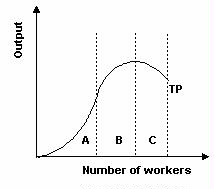### CFA Practice Question

There are 923 practice questions for this topic.

### CFA Practice Question

Refer to the graph below. Within which section(s) of the production function is marginal product decreasing?A. A
B. B
C. B and C

The slope of the production function is decreasing in ranges B and C, so marginal product is falling.

User Comment
chuong Why???. Look at B section, TP is increasing at decreasring rate and in C section, TP is decreading at increasing rate. can someone explain???
RichWang Marginal product is failling in both B and C. It has faillen into negative in C, but that's still failling.
rana1970 look carefully in section B, each additional worker is making comparatively less output, so the answer. look in another way, if we move on the curve upward in section B,the slope of tangential line keep on decreasing and become zero while entering in section C, meaning that marginal output(additional output) is decreasing.
myzec Remember the graph shows total product. The question asks where marginal product is decreasing. Thus we find the change in marginal product by looking at the slope of Total product. When you have no figures you look at the shape of the graph. In section A we have a convex shape indicating the slope is positive ie marginal product is increasing. In section B and C we have a Concave shape indicating that marginal product is decreasing. In both section A and B however we see that Marginal product is positive. Remember we are not looking to see if marginal product is positive or negative we are looking for the slope of marginal product.
jerylewis marginal = SLOPE
ljamieson convex region
magicchip S L O P E !!!
Jolen you have to look at the slope to get marginal product. the graph shows the TP line so it can be confusing...
zulfi79 myzec , makes sense...I like this comments thing with questions.
erinelize Thank you, myzec!
aragarwal myzec thanks
Huricane74 Please correct me if I am wrong, but this is how I interpret the graph:
In A, marginal product is increasing, and increasing at an increasing rate.
In B, marginal product is increasing, but increasing at a decreasing rate.
In C, marginal product is decreasing.